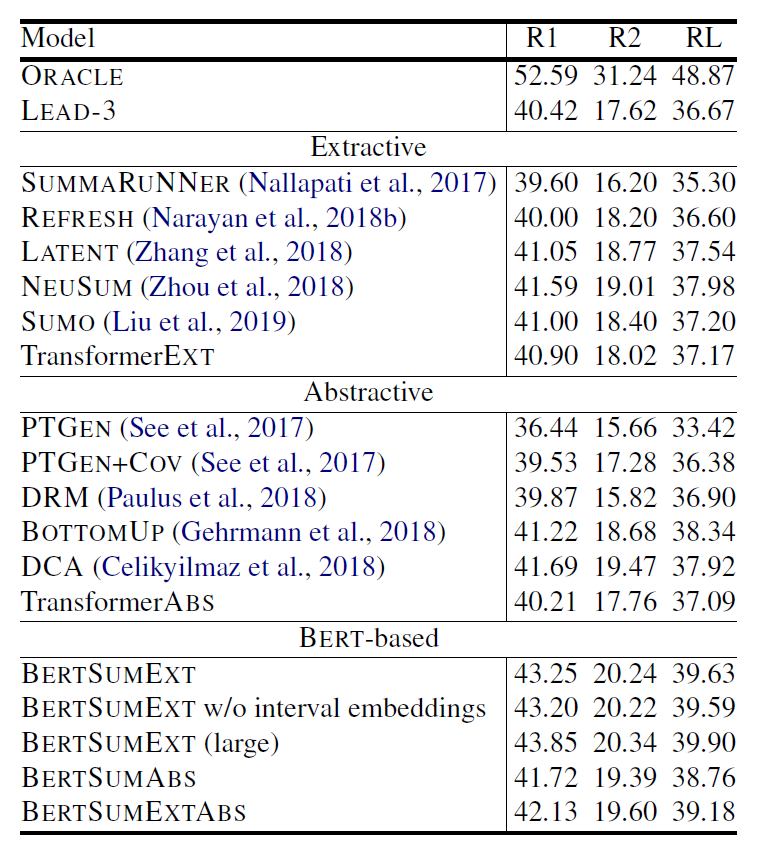## Abstract

1. 分析 document encoding 對於 summarization task 的重要性
2. 提出如何有效地應用預訓練模型在不管是 extractive 或 abstractive 下的 summarization task

## Background

### BERT

BERT 這個由 Google 在 2019 所提出的模型相信大家都不陌生，在提出後就刷了各種任務的榜，成了 NLP 領域無人不知無人不曉的模型。論文中對 BERT 的輸入進行了修改，讓其更好的對應 summarization task 的特性，為了對比，筆者先複習一下原版的 BERT 的輸入。

\widetilde{h}^l = LN(h^{l-1} + MHAtt(h^{l-1}))
h^l = LN(\widetilde{h}^l+ FFN(\widetilde{h}^l))

h 是 hidden states，h^0 = X；LN 是 Layer normalization；MHAtt 是 multi-head attention；l 是疊幾層 Transformer Layers 的層數。最終 BERT 會輸出 T = [t_1, t_2, \dots , t_n]，每個 t_i 是擁有語意資訊的 word embedding。

### Extractive and Abstractive Summarization

extractive summarization 為藉由在文章裡找尋重要的句子，並把找出來的句子接在一起，當作這篇文章的 summary。在模型訓練上，則是當做 sentence classification 的任務，藉由分類每個句子是否為重要句子，來得到要做為 summary 的句子。

abstractive summarization 則是將原始文章餵給模型吃，並直接吐出一段 summary 的方法，是一個 sequence to sequence 的問題。abstractive 相較 extractive 困難的點為，abstractive 需要能夠產生原本不存在於文章的詞彙或句子，並且要符合語言的規則。

## Fine-tuning BERT for Summarization

### BERTSUMEXT：BERTSUM of Extractive Summarization

\widetilde{h}^l = LN(h^{l-1} + MHAtt(h^{l-1}))
h^l = LN(\widetilde{h}^l+ FFN(\widetilde{h}^l))

h 是 hidden states，h^0 = PosEmb(T)T 代表 BERTSUM 輸出的 sentence embeddings。

\hat{y}_i = \sigma(W_0h_i^L+b_o)

h^L_i 就是 Transformer第 Lsent_i 的輸出。

## Result表（2）ROUGE F1 在 CNN/DailyMail test set 的表現 (R1 and R2 are shorthands for unigram and bigram overlap; RL is the longest common subsequence)

## Introduction

SimCSE 關注的問題是 universal sentence embeddings，這在 NLP 領域是個基礎且重要的問題。SimCSE 提出一個應用 Contrastive Learning 的訓練方法，來訓練預訓練語言模型（如：BERT 或 RoBERTa），可以使用不管是 unlabeled 或 labeled data 來訓練 sentence embeddings。在使用 unlabeled data 進行 unsupervised learning 的情況下，SimCSE 在 STS（Semantic textual similarity）任務的表現上，不只是遠超其它之前提出的 unsupervised models，甚至能超越 supervised models，使用 labeled data 進行 supervised learning 則更不用說了，穩穩的成為 STS 任務的新 SOTA。如果將 SimCSE 訓練出的模型，遷移到其它任務進行微調，則表現也能比之前提出的其它方法有相當抑或好上一點的表現。

• Contrastive Learning
• Unsupervised SimCSE
• Supervised SimCSE
• Result
• Conclusion

## Unsupervised SimCSE

Unsupervised SimCSE 最關鍵的地方就是對如何建構 positive pair 的回答，對於 CV 領域有很多方法，如：cropping、flipping、distortion、rotation 這些 augmentation method，NLP 在近年也有許多方法被提出，如：word deletion、reordering、substitution。但作者發現這些方法反而會傷害到模型的表現，對此作者最後給出的答案非常簡單，那就是 dropout，dropout 可以作為 minimal data augmentation，並達到比之前其它的 augmentation 方法更好的表現。

## Conclusion

SimCSE 提出利用 Contrastive Learning 進行訓練，可以使用不管是 Supervised 或 unsupervised learning 進行訓練，方法簡單暴力，在 STS 任務上成為新 SOTA。

## Mixed Precision Training（Pytorch 範例程式）

Mixed precision training 是由百度與 NVIDIA 發表於 ICLR 2018 的論文，其提出一種訓練方法，使得在使用 half-precision(FP16) 訓練模型的情況下，也能達到與使用 single-precision(FP32) 訓練相當的表現，且得到加快訓練速度與降低顯存需求的效果。

## Motivation

• arithmetic bandwidth
• memory bandwidth
• latency

memory bandwidth 的壓力會降低，因為我們只需要更少的 bits 來存儲同樣的參數。

## Implementation

• fp32 master copy of weights
• loss scaling
• arithmetic precision

### Loss Scaling

loss scaling 的想法很簡單，既然大多數 gradient 都很小，超過了 FP16 的表示範圍，那就乘上一個值讓它先變大，在更新時再縮回來不就好了。

## Conclusion:

1. 使用 FP32 存 master copy of weights
2. 在每次疊代
1. copy 一個 FP16 的 weights
2. Forward propagation（FP16 weights and activations）
3. 把 loss 乘上 scaling factor S
4. Backward propagation（FP16 weights, activations, and their gradients）
5. 把 weight gradient 乘上 1/S
6. 完成 weight 的更新

## Code

import torch
import torch.nn as nn
import torch.optim as optim
import torch.utils.data as dset
from torchvision import datasets, transforms, models

# create transform
transform = transforms.Compose(
[transforms.ToTensor(),
transforms.Normalize((0.5,), (0.5,)),]
)
# Data
# Model
class Model(nn.Module):
def __init__(self):
super(Model, self).__init__()
self.base = nn.Sequential(
nn.ReLU(inplace=True),
nn.MaxPool2d(kernel_size=2),
nn.ReLU(inplace=True),
nn.MaxPool2d(kernel_size=2),
)
self.fc = nn.Linear(32*7*7, 10)

def forward(self, input):
feature = self.base(input)
feature = feature.reshape(feature.size(0),-1)
output = self.fc(feature)
output = nn.functional.log_softmax(output, dim=1)
return output
model = Model().to('cuda:0')

optimizer = optim.SGD(model.parameters(), lr=1e-3)
criterion = nn.NLLLoss()
﻿

for epoch in range(10):
input, target = input.to('cuda:0'), target.to('cuda:0')